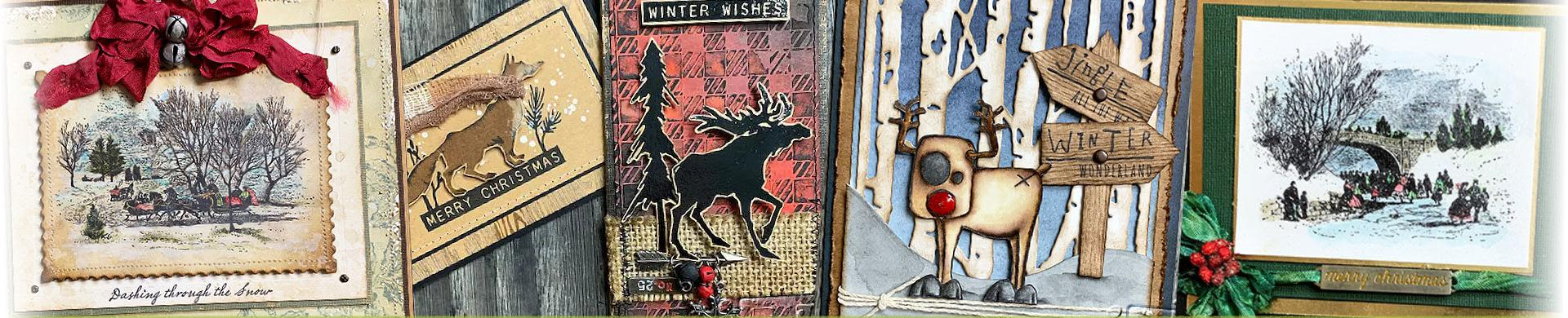# Tim Holtz Rubber Stamps - ChristmasCreate unique Christmas cards and home decor makes with Tim Holtz deep etched red rubber stamps.
• Brand
1. \$28.91
2. \$24.09
3. \$24.09
4. \$24.09
5. \$28.91
6. \$28.91
7. \$24.09
8. \$28.91
9. \$24.09
10. \$28.91
11. \$24.09
12. \$28.91
13. \$24.09
14. \$24.09
15. \$24.09
16. \$24.09
17. \$24.09
18. \$24.09
19. \$24.09
20. \$28.91
21. \$28.91
22. \$28.91
23. \$28.91
24. \$28.91
25. \$28.91
26. \$28.91
27. \$28.91
28. \$28.91
29. \$28.91
30. \$24.09
31. \$28.91
32. \$24.09
33. \$28.91
34. \$28.91
35. \$24.09
36. \$28.91
37. \$28.91
38. \$28.91
39. \$28.91
40. \$24.09
41. \$28.91
42. \$24.09
43. \$24.09
44. \$24.09
45. \$28.91
46. \$24.09
47. \$28.91
48. \$24.09
49. \$28.91
50. \$24.09
51. \$24.09
52. \$28.91
53. \$24.09
54. \$24.09
55. \$24.09
56. \$24.09
57. \$24.09
58. \$28.91
59. \$24.09
60. \$24.09
61. \$24.09
62. \$28.91
63. \$28.91
64. \$24.09
65. \$24.09
66. \$24.09
67. \$28.91
68. \$28.91
69. \$24.09
70. \$24.09
71. \$24.09
72. \$24.09
73. \$24.09
74. \$24.09
75. \$24.09
76. \$24.09
77. \$24.09
78. \$28.91
79. \$24.09
80. \$24.09
81. \$24.09
82. \$24.09
83. \$28.91
84. \$24.09
85. \$28.91
86. \$24.09
87. \$28.91